﻿ Optimal Design of PID Controller for an AVR System Using Flower Pollination AlgorithmPublications are Open
Access in this journal
Article Versions
Export Article
• Normal Style
• MLA Style
• APA Style
• Chicago Style
Research Article
Open Access Peer-reviewed

Optimal Design of PID Controller for an AVR System Using Flower Pollination Algorithm

D. K. Sambariya , Tripti Gupta
Journal of Automation and Control. 2018, 6(1), 1-14. DOI: 10.12691/automation-6-1-1
Published online: March 17, 2018

Abstract

In this presented work, terminal voltage and reactive power control at the different conditions of load changes of synchronous generator in the AVR system is presented. To improve the step response of the alternator terminal voltage proportional integral derivative controller is carried out with optimization technique as Flower Pollination Algorithm (FPA) to optimally tuned the parameters of PID controller. The AVR system response with proposed FPA-PID controller is found to be better and compared that of with controllers in literature (42 in number). The performance is compared in terms of peak value, settling time and performance indices like ITAE, ISE and IAE. The robustness analysis of the proposed FPA based PID controller is considered for 156 plant conditions and varying time constants parameters as ±20% system conditions. The system stability with proposed FPA optimized PID controller also analysed in the form of eigenvalue, damping factor and frequency of oscillations.

1. Introduction

To deliver the efficient, economically and reliably maintained electrical power supply to the load and consumers, the stability and performance of synchronous generator also improves in the power system network or power stations 1. The synchronous generator has three main control systems such as exciter, governor and boiler. The objective of these control systems has to consistent a voltage and current by excitation system, steam pressure in the boiler by firing control and third one is control the torque speed of turbine by governor 2.

In this work, excitation system is considered which includes exciter and (AVR) Automatic Voltage Regulator. The aim of this control strategy is to deliver and economically maintain a generator terminal voltage at specific level. AVR is a device to control the variation of terminal voltage of synchronous generator at certain level under system uncertainties such as fault and load fluctuations. Due to this, steady-state error can take place in the uncontrolled AVR system 3. To overcome this problem, PID controller is constructed because of it is robust and simple in structure.

Many optimization techniques have been developed such as classical methods, intelligent control techniques and soft-computing techniques. In the classical methods, Cohen-Coon (CC), TyreusLuyben (TL), Internal Model Control (IMC), Zeigler-Nicolas (ZN), Chien-Hrones-Rewich (CHR) method etc. are considered. Fuzzy Logic controller (FLC), Artificial Neural Network (ANN), Multilayer Perceptron Neural network (MLPNN), Neuro-Fuzzy controller etc. have considered in the Artificial intelligent techniques. In the categories of soft-computing techniques, different metaheuristic or nature inspired tuning techniques have been taken into account for example Hybrid Evolutionary Algorithm (HEA), Genetic Algorithm (GA), Particle Swam Optimization (PSO), Local Unimodal Sampling (LUS) algorithm etc.

Rao et al., 2014 4 demonstrates a design of Internal Model Control (IMC) based PID controller cascade with lag-lead filter with modified IMC filter to provide load disturbance rejection for the first order process system. Mittal and Rai, 2016 5 describes the performance of conventional controller for AVR system and presents the simulation results with IMC controller, PID controller and cascade controller. Bansal et al., 2012 6 have explain a review on classical and intelligent computational techniques for tuning the PID controller such as ZN, CC, ACO, GA etc. are considered.

Artificial Neural Network (ANN) based MLPNN-AVR system and Feed Forward ANN (FFANN) based AVR system is presented by Memon 1 in respectively and improve the transient stability of synchronous machine. Shayeghi et al., 2015 7 have described FLC based Fuzzy P + Fuzzy I + Fuzzy D (FP + FI + FD) controller and controlled the generator terminal voltage in the AVR system. For optimal tuning and robust performance Hybrid GAPSO optimization technique is used and comparisons are made with conventional PID and fuzzy PID controller.

Wong et al., 2009 8 design a HEA which is a combination of GA and PSO technique to applied PID controlled AVR system and show that proposed new fitness function gives optimal and effective response. Mohanty et al., 2014 3 presents the LUS algorithm to design PID controller for AVR system with modified objective functions and perform the simulation in terms of stability, settling time and peak overshoot.

In this research work, FPA based PID controller have designed to applied on AVR system and increase the effectiveness of the system response. The FPA is bioinspired and reproduction based optimization technique in which pollen is transferred from one flower to another flower through pollinators such as butterflies, bees and animals, known as pollination in the flower plants. Self-pollination and Cross-pollination are two types of pollination. The objective of this algorithm is the most fittest and optimal reproduction of flowering plants.

2. Description of AVR System Modeling

As Discussed previously, that AVR maintains the system voltage to its nominal value and measure this regulating voltage by sensor and again fed to the synchronous generator 9, 10. The AVR system consists for components defined by exciter, amplifier, generator and sensor. The general model of AVR system is shown in Figure 1.

• Figure 1. General model of AVR
2.1. Mathematical Modelling

The components of AVR represented by transfer function to demonstrate the dynamic performance of the system. The AVR system without and with PID controller are shown in Figure 2 and Figure 3, respectively. The used value of parameters of transfer function model such as gain and time constants are mentioned in Table 1. The mathematical modelling of AVR system is as follows:

• Figure 2. Model of AVR without controller
• Figure 3. Model of AVR with controller

2.1.1. Amplifier

The model of amplifier in terms of gainand time constant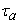are mathematically represented as in Eq. 1. The range of time constant and gain are considered as 10 to 400 and 0.02 to 0.1 seconds, respectively.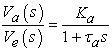(1)

2.1.2. Exciter

The transfer function model of exciter system shown in Eq. 2. The limits 1 to 400 is taken for exciter gain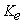and 0.25 to 1.0 second is assumed for time constants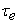.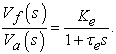(2)

2.1.3. Generator

The Eq. 3 demonstrates the transfer function model in the form of gain and time constants. The range of time constants may vary between 1.0 to 2.0 seconds and gain may vary between 0.7 to 1.0.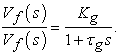(3)

2.1.4. Sensor

The mathematical equation of sensor model is denoted by Eq. 4. The set value of limits of time constants is 0.001 to 0.06 seconds and set value of limits of gain is 1.0 to 10.0.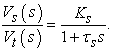(4)
2.2. PID Controller

The PID controller is a mechanism of control loop feedback and most commonly used controller to improve the quality of response of AVR system 11. It has a short rise time i.e. fast speed of response and higher stability. It is a combination of three controller modes such as Proportional, Integral and derivative mode. In the first mode, if the value of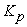(proportional gain) changed in the increasing mode then steady-state error and rise time reduced. However, after certain limit the increasing value ofproduced overshoot and system will be unstable 12, 13.

Similarly, by enhance the value of integral gain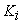, rise time also decreased with eliminating steady-state error. Whereas, after specific limit increase overshoot with worse transient response by using raise value of integral gain. In the derivative mode, gainmay vary in the increasing manner then value of settling time and overshoot decreased with improved stability of the system 14.

• Figure 4. Block diagram of PID controller

The block diagram of PID controller with FPA optimization technique is presented in Figure 4. The s-domain transfer function represented by Eq. 5 and time domain output is denoted by Eq. 6 of PID controller.(5)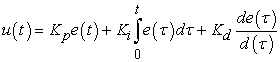(6)

Where the error signal is denoted by e(t) and the control signal is shown by u(t). the tuning parameters,,. are mostly required to design the PID controller.

2.3. Objective Function

In this research work, the design of PID controller is considered as problem of optimization. The objective function is evaluated as minimization of error signal as in Eq. 7 with parameters limits as in Eq. 8.

Minimize: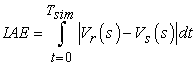(7)

Subjected: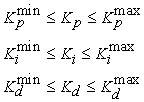(8)

3. Flower Pollination Algorithm

The nature inspired and biological system based optimization techniques are current trend to solve complex constraints and difficult problems in terms of optimal solution in engineering and industrial applications 15. The FPA is a bio inspired algorithm which mimics the behaviour of pollination process of flower plants in the nature 16. It is recently introduced by Xin-She Yang in 2012. The objective of flower plants is eventually reproduction through pollination.

3.1. Characteristics of bio-inspired FPA

The bio-inspired FPA is related with the process of pollination. In the process of pollination, pollen is transferred through pollinators such as butterflies, bees, insects and other animals 17. In really, specific flower plants and insects have evolved together into a flower pollinator partnership. For example, some specific flowers are dependent on particular species of birds, insects, for actual process of pollination 18.

The pollination process in flowering plants is divided into two categories: Biotic and Abiotic. When pollen is transferred by insects, birds, butterflies, bees and animals, it is known as biotic pollination and about 90% in the nature 19. If pollinators are not required to transform pollen then it is called as abiotic pollination and about 10% in the nature 19. In such type of flowering plants, pollination can take place with the help of wind and diffusion. Grass is a good example of abiotic pollination. The types of pollination are represented in Figure 5.

Honeybees are the best pollinator in the nature because of they can develop flower constancy or pollinator constancy. That is, pollinator constancy is demonstrate as, the movement of particular pollinators to completely visit specific flower species though avoiding other species of flower plants 18. Such pollinator constancy may have more beneficial as a consequence of this will increase the reproduction of same species of flower plant by transferring pollen to the nonspecific plant of flower 17.

The process of pollination can take place with the help of cross-pollination and self-pollination. Cross-pollination (allogamy) occur when pollen is transferred from one flower of a plant to another flower of different plant. However, self-pollination can take place when pollen transferred to the same flower or another flower of same branch, which frequently occur when reliable pollinator is not available 16. Self and cross-pollination are represented in Figure 6.

• Figure 5. Types of pollination
• Figure 6. Self and cross pollination

Biotic, cross-pollination is considered as the global pollination, in which pollinators such as bees, butterflies and other flying insects transferred pollen from one flower to another at a long distance and these pollinators may behave as Lѐvy flight with fly distance steps obey a Lѐvy distribution 17. Moreover, flower constancy can be used an increment steps using the similarity or difference of two flowers.

3.2. Mathematical Modelling of FPA

According to above characteristics of process of pollination following rules can be followed by the pollinators 20:

When pollinators transferred pollen by performing Lѐvy flight with process of global pollination then it is considered as biotic and cross-pollination.

Local pollination is coming into the categories of abiotic and self-pollination.

The same properties of two flowers are proportional to the probability of reproduction, it can be considered as pollinator constancy.

Switch probabilityis used to control the process of local and global pollination. In the whole process of pollination because of physical proximity and other factor such as wind.

Based on the discussion of above characteristics of pollination process, The FPA optimization technique have developed, therefore, convert the above four rules in the form of mathematical modeling equations.

In the first step of global pollination, pollen gametes are transferred through pollinators such as flying insects can travel over a long distance. This process ensures that pollination and reproduction of the fittest solution define as f*. The rule 1 and flower constancy can be expressed as Eq. (9) 15.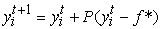(9)

Wheredefine the pollen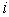or vector of solution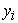at generation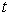. The symbol f* presents the current optimal solution at current no. of iteration that is found among all the solution. Strength of the pollination represented by element P, which basically a step size.

The flying insects may travel over a long distance while transfer pollen so we can define this characteristics in terms of Lѐvy flight. That is,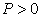illustrated from a Lѐvy distribution 15.(10)

In Eq. (10) standard gamma function is represented by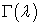and this type of distribution is applicable for large steps. Considered value ofis 1.5.

Equation (11) explain the local pollination and rule 2 plus flower constancy can be mathematically modelled as 15: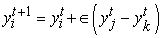(11)

Where pollen of different flowers of same plant shown by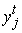and. This basically mimics the flower constancy in a limited neighbourhood. Ifand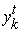belongs to the same species and same population, this become a local random walk if we describefrom a uniform distribution in the range [0,1] 15.

Mostly, at all scales, both global and local, activities of flower pollination can occur. In practices, adjacent flower patches are more likely to be pollinated by local flower pollens that those far away. Due to this, we can follow fourth rule (switch probability) p to switch between common global pollination to intensive local pollination. The pseudo code of the algorithm as following: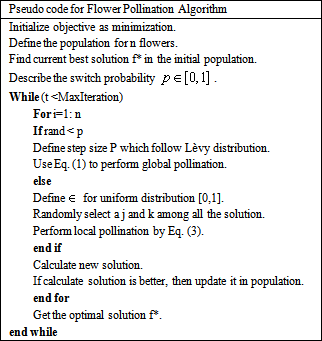• Figure 7. Flowchart of FPA

If we consider p=0.5 as initial value and find the most appropriate parameters range through parametric study. We can consider p=0.8 for better response for most of the applications. Algorithm for FPA shown below 13 and flow chart also presented in Figure 7.

4. Result and Discussions

4.1. Performance of FPA

The proposed PID controller tuning parameters are determined by application of optimization using FPA with sampling time 0.02 sec. The problem of optimization is considered as minimization of integral absolute error as defined in Eq. 7. The Simulink model of AVR system with global variables of PID controller as,andis synthesized in MATLAB R2011b as shown in Figure 4. The IAE based objective function as in Eq. 7, with global variables is written in *.m file in MATLAB 21. The upper and lower bound to the PID parameters are set as shown in Eq. 12. The Number of population size to FPA are considered as 10 and the dimension is set to 3. The switch probability is considered as p=0.8 and the maximum number of iteration count is set as 100 for considering the termination of optimization. The performance of optimization in terms of fitness function value and the variations of PID parameters with iteration count is set as 100 and sampling time set as 0.02 second during optimization is shown in Figure 8(a) – Figure 8(d).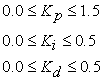(12)
4.2. PID Controller in Literature

In this section, the PID controllers using different techniques in literature are to be reviewed. The detail of the PID parameters with system parameters is enlisted in Table 2.

Afroomand, 2016 has considered the three variant of Vector Based Swarm Optimization (VBSO) based on mutation as well as selection of weighting coefficients and off-springs. The three variants of VBSO are represented by VBSO-1, VBSO-2 and VBSO-3 19. The VBSO variants perform well in terms of accuracy and speed of convergence; however, the VBSO-2 variant performs better with respect to other variants. Therefore, the data of best-performing variant, i.e. VBSO-2 is considered for comparison purposes and enlisted in Table 2.

Chatterjee, 2016, has presented the application of the Teaching Learning Based Optimization (TLBO) tuning of PID parameters in AVR system under generator parametric variation. It presented the dynamic behavior of generator and application of TLBO to control the system behavior through PID controller. The different PID controllers for different sets ofand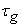are presented as enlisted in Table 2 20.

The application of Gray Wolves Optimizer (GWO) for tuning the PID parameters is presented in 21. In the GWO, three levels of hierarchy are considered which is represented by α, β and δ. The last level of the hierarchy is ω. The parameters of PID controller tuning using (GWO) and conventional PID parameters are included in Table 1 21.

The design and performance analysis of PID controller using multi objective non-dominated sorting genetic algorithm-II (NSGA-II) with application of AVR system is presented in 22. The effect of cost function and PSO is considered for optimal design of PID parameters in 23. The priority based objective function for minimization of ISE for tuning the PID parameters using Gravitational Search Algorithm (GSA) is shown in 24. The CPSO is considered in 25. The application of TLBO is as well considered in 26. The Taguchi combined Genetic Algorithm (TCGA) based designed parametric values of PID controller for AVR system is presented in Table 2 27.

The PID controller design using ZN, PSO, and Many Optimization Liaisons (MOL) are designed in 28. The GA and PSO are considered for optimal tuning of PID parameters of an AVR system in 29. The different values of the weighting factors are considered as 0.5 and 1.0 at different generations in 29 and included in Table 2. The PID controller tuned with ZN method is included in Table as presented in 30. The application of Anarchic Society Optimization (ASO) for optimal design of PID control of an AVR system and comparing the results with VEPSO and CRPSO under varying conditions of and for five cases. These value are varied to analyze robustness of the proposed control strategy in 31. The MOL is considered for optimal design of PID controller in 11, 32.

The Pattern Search Algorithm (PSA) for tuning the PID parameters is presented in 33. Mandal, 2011 has presented Memetic Algorithm (MA) to find optimal PID parameters for AVR system. It is explained by combining a competitive variant of differential evolution (DE) and a local search method. It presents a competitive variants of DE is used for global exploration and the classic soils Wet’s algorithm (cDESW) is used as a local search process. PID parameters of this algorithm at different number of generations and different values of alpha are shown in Table 2 34. The application of PSO is revisited in 35 for designing the optimal values of PID parameters. Madinehi et al., 2011 have presented the application of Shuffled Frog Leaping (SFL) algorithm and PSO for determining the optimal PID parameters 36.

The application of chaotic ant swarm (CAS) algorithm based PID controller for AVR system is presented in 37. The Lozi map based chaotic optimization approach is used in 12 for application of AVR system. The craziness based PSO (CRPSO) binary coded GA are the two props used to evaluate the optimal PID gains. Under nominal operating conditions, CRPSO proves to be more robust than GA in performing optimal transient performance. The PSO optimization approach for determining optimal PID parameters of an AVR system with two different set of are considered in 38.

• Figure 8. The behavior of FPA in terms of fitness function, PID parameters and iteration count
4.3. Simulation Results

The transient response performance is compared and presented in Table 3. It represents the transient response comparison in terms of peak value and settling time with set value of sampling time is 0.02 seconds for the different PID controllers as demonstrate in the literature. The performance of Flower Pollination Algorithm based PID controller (FPA-PID) controller in terms of peak value and settling time is better as compared to other PID controllers in literature. The peak value and settling time of the system response with proposed FPA-PID is 0.9976 and 1.448 second, respectively and is mentioned in Table 3.

Afroomand, 2016 19 generates the step response in terms of peak value and settling time with the help of VBSO-2 technique and values are 1.119 and 4.613 sec, respectively which is greater than the proposed FPA-PID controller. In Chatterjee, 2016 20; the TLBO technique is used to design the PID controller for varying generator parameters and presented the values of peak as 1.126, 1.137, 1.123, 1.129 and settling time as 4.690 sec, 4.575 sec, 4.747 sec, 4.804 sec, respectively as shown in Table 3. All the values of peak and settling time are also high in comparison to proposed FPA optimization technique.

Verma, 2015 21 presents the Conventional PID, GWO and GWO technique for AVR system and evaluates the peak with values 1.648, 1.118, 1.117 and settling time with values 6.044, 4.727, 4.632, respectively. Yegireddy, 2015 22 considered NSGA-II algorithm and evaluate the transient response in terms of peak value and settling time as 1.211 and 5.817, respectively. Aberbour, 2015 23 represents the PSO-PID controller and calculate the peak value is 1.181 and settling time is 3.983 seconds.

The GSA, CPSO, and TLBO techniques are presented in 24, 25, 26, respectively with system response as peak value and settling time of generator terminal step response as shown in Table 3. Hasanien, 2013 27 presents the three values of peak and settling time for three different values of PID parameters as 1.152, 1.161, 1.167 and 4.250, 4.117, 4.097, respectively, using TCGA method.

Nirmal, 2013 28 represents the three methodologies throughout the article are ZN, PSO, and MOL and get the values of peak 1.642, 1.307, 1.144 and settling time with values 3.803, 4.651, 4.212, respectively. Kumar, 2013 29 presents the use of PSO and GA technique at different values of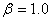and, respectively. The step response comparison in terms of peak values and settling time are given by 1.100, 1.108 and 5.988 secs, 5.912 secs, respectively.

Bayram, 2013 30 obtained peak value is 1.488 and settling time is 3.391 secs, which are designed using the ZN method. Shayeghi, 2012 31 presents the controllers for AVR system using ASO, VEPSO, and CRPSO techniques with generator parametric variations. The response parameters using these controllers as peak values and settling time are 1.082, 1.082, 1.065 and 6.256, 6.275, 6.218, respectively.

Sahu, 2012 32, Panda, 2012 11 and Sahu, 2012 33 gives the values of peak as 1.097, 1.118, 1.126 and settling time as 5.358, 4.938, 5.110 using optimization technique MOL, MOL(ITAE) and PSA, respectively. Mandal, 2011 34 performs the cDESW technique at the value of weighting factor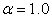and. The PID controller based on cDESW, gives response with peak value and settling time as 1.180 and 4.078 secs., respectively. However, the PID controller using cDESW, presents peak value as 1.131 and settling time as 4.728 secs 34.

Rahimian, 2011 35 uses the application of PSO algorithm for designing the PID controller and the response presents peak with value 1.136 and settling time with value 4.594 sec. Madinehi, 2011 36 obtain a values of peak and settling time by using SFL and PSO optimization techniques with values 1.019, 0.9863 and 6.008 sec, 1.519 sec, respectively as enlisted in Table 3. Zhu, 2009 37 have presented the performance in peak value and settling time as 1.114 and 5.530 secs, respectively with the help of CAS-PID controller. In Dos, 2009 12 perform the COLM technique for parametric variations of generator as shown in Table 3 and produces a peak with values 1.131, 1.103, 1.087, 1.127, 1.092, 1.078 and settling time with values 4.785 sec, 5.855 sec, 6.943 sec, 4.996 sec, 6.065 sec, 6.848 sec, respectively as mentioned in Table 3. Gaing, 2004 38 consider PSO algorithm for different weighting factors and values and results with peak values and settling time as shown in Table 3.

• Figure 9. The sample response comparison of the AVR system with proposed PID controller (FPA-PID) and controllers in literature [11,23,24,28,30,35]

4.3.1. On Performance Indices

The step response of the AVR system is discussed in terms of performance indices like ISE, IAE and ITAE as well described below in Eq.13 – Eq. 15.(13)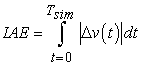(14)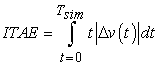(15)

4.3.2. Comparison of PIs

The analysis of the performance of PID controller design for the AVR system with different types of performance indices like Integral Square Error (ISE), Integral Absolute Error (IAE), and Integral Time Absolute Error (ITAE) as discussed in section 4.3.1 the minimum value of performance as compared to others. In Table 4, the evaluated performance indices as ISE, IAE and ITAE for proposed algorithm is 19.5332, 19.6362 and 199.8672, respectively. These values are lesser as compared to all other optimized controllers as presented in Table 4 and all these values are measured at simulation time 20 seconds and sampling time 0.02 seconds.

4.3.3. Based on ITAE

Madinehi, 2011 36 gives better performance to other controllers in literature except proposed in terms of ITAE for PSO-PID controller with value as 199.8733. The ITAE value for ZN-PID controller in 28 is 200.1208. Verma, 2015 21 presents the ITAE value as 200.1462 for conventional PID controller and higher in comparison to proposed resulting the poor performance. These controllers are poor in performance as compared to proposed but better to ZN-PID 30, PSO-PID 28 and SFL-PID 36 controllers with ITAE values 200.1764, 200.3359 and 200.3392, respectively.

On comparing ITAE in Table 4, the worst performing controllers are Gozde,2014 25 for CPSO technique, Dos, 2009 12 for COLM technique with generator parameters (and), Kumar, 2013 29 for different weighting factors using PSO and GA optimization technique with values as 201.2018, 200.7798, 200.7106, 200.6973, 200.6437, respectively.

4.3.4. Based on IAE

In this analysis, according to the best solution in Table 4, for IAE error function, only Madinehi, 2011 36 for SFL-PID and PSO-PID controller obtained a best value as 19.6177 and 19.6087, respectively comparison to proposed FPA-PID controller with value as 19.6362 but all PID controllers in literature gives poor performance in comparison to proposed method.

According to the best solution, Shayeghi, 2012 31 and Sahu, 2012 32 appears to be fourth and fifth priority with IAE values 19.7100, 19.7287, using CRPSO and MOL optimization technique respectively. Similarly, Zhu, 2009 37 for CAS-PID controller with value of IAE as 19.8552.

The worst performing controllers are based on ZN-PID and conventional PID controllers in 21, 28 with IAE values as 19.9594 and 19.9237, respectively. the other controllers in Bayram, 2013 30, Gozde, 2014 25, Sahu, 2012 33, presents the IAE values as 19.9191, 19.9142, 19.8919, respectively.

4.3.5. Based on ISE

In this analysis, according to the best solution, PSO-PID controller in 36 obtains a best value as 19.4970 comparison to proposed method with ISE value as 19.5332. However, all remaining PID controllers in literature gives poor performance as compared to proposed FPA optimized PID controller.

The SFL-PID controller 36, CRPSO-PID controller 31, TLBO-PID controller 26 with ISE values 19.5415, 19.7631, 19.7814, respectively, may be considered with third, fourth and fifth priority in selection of a controller as compared to controllers in literature and proposed one. However, the controller based on MOL optimization technique may be considered on sixth priority with ISE value as 19.8243 32.

The worst performing controllers are verma, 2015 21, Gozde, 2014 25, Nirmal, 2013 28 with ISE value as 20.3968, 20.3461, 20.3210, respectively. Similarly, Bayram, 2013 30 and Yegireddy, 2015 22 for ZN-PID controller and NSGA-II method presents the ISE values as 20.2376, 20.1439, respectively.

4.3.6. Eigenvalue Analysis

In this section, eigenvalue analysis is performed in terms of oscillatory modes, damping factor and frequency of oscillations for proposed FPA and PID controllers in literature with sampling time 0.02 seconds.

4.3.7. Based on Oscillatory Modes

In the analysis of oscillatory modes, proposed FPA optimized PID controller obtained better stability in comparison as compared to TCGA method 27, COLM technique 12 for generator parameters such asand,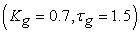NSGA-II method 22, VBSO-2 method 19, ZN-PID controller 30, PSA-PID controller 33, Conventional PID controller 21 as presented in Table 5.

The worst performing controllers which are lies in the right half of s-plane and moves the system towards unstable region is ZN-PID controller 28 has first priority. Similarly, TLBO-PID controller 26, CPSO-PID controller 25 and COLM technique 12 have second, third, fourth priority, respectively as shown in Table 5.

4.3.8. Based on Damping Factor

The proposed FPA-PID controller calculate the value of damping factor is 0.0750 which is better because of oscillations damped out early in comparison to COLM technique 12 for varying generators parameters such as,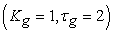,andpresents the values of damping factor as 0.0722, 0.0691, 0.0309, -0.0025, respectively.

The NSGA-II method 22, VBSO-2 method 19, ZN-PID 30, Conventional PID 21, PSA-PID 33, CPSO-PID 25, TLBO-PID 26, ZN-PID 28 controllers obtained the values of damping factor as 0.0675, 0.0507, 0.0230, 0.0053, 0.0047, -0.0114, -0.0412, -0.0733, respectively as enlisted in Table 5.

4.3.9. Based on Frequency of Oscillations

In this analysis, proposed FPA optimized PID controller has frequency of oscillations with value 0.7588 which is better in comparison to Dos, 2009 12 for generator parametersand. The PSA-PID controller 33, CPSO-PID controller 25, TLBO-PID controller 26 also gives worst performance in comparison to proposed method with values of frequency of oscillations as shown in Table 5.

4.4. Robustness Performance

Two conditions have analyzed to observe the stability and robust performance of the AVR system such as (a) The eigenvalue analysis with varying generator parameters (gain and time constants). (b) The time domain analysis with varying time constant parameters of AVR system for ± 20% conditions.

4.4.1. Eigenvalue Analysis for 156 Plants with FPA-PID Controller

In the literature, AVR system models represented based on changes in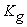andas shown in Table 2. The four plant conditions are considered in 20 from the range of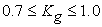and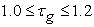. Dos 2009 12 have analyzed six plant conditions ofandfrom the range ofand, respectively. In this paper, the generator parameters are subjected to change with step size of 0.10 within the range of parameters as shown in Eq. 16. The combined effect of these parameters obtained 156 plant conditions. The eigenvalue plot of the AVR system for different 156 plant conditions with proposed FPA-PID controller is shown in Figure 10.

• Figure 10. Eigenvalue plot for 156 plant conditions with proposed PID controller to AVR system

It can be seen that none of the eigenvalue is lying in right hand side of the s-plane. Therefore, the system is stable for these 156 plant conditions and providing to be robust in operation in terms of stability with proposed FPA optimize PID controller for such wide range of plant conditions.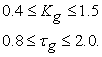(16)

4.4.2. Performance with Varying AVR System

Robustness analysis of any power system is very necessary because of power system operating point varies with different values of load and also the system parameters are not constant 20. In the presented work, to analyze the robustness of the AVR system with FPA optimized PID controller with sampling time set as 0.02 second, the uncertainties of the AVR system described in terms of varying time constants parameters of amplifier, exciter, generator and sensor between the range of -20% to +20% in steps of 5% for maintaining the actual response of the system in abnormal cases and observe the behavior of the AVR system.

The behavior of the AVR system with varying time constants along with the actual response is shown in Figure 12 to Figure 15. The robustness of the system performed by evaluating settling time, peak amplitude and PI’s with ISE, IAE, ITAE as enlisted in Table 6. The open loop response of AVR system shown in Figure 11 produces the settling time and peak with values 8.542, 1.506, respectively. The values of ISE, IAE and ITAE with values 16.7520, 18.0661, 181.8482, respectively as shown in Table 6.

• Figure 11. Response of AVR system without controller
• Figure 12. Robustness analysis of AVR system by changing time constant ranging from -20% to +20% for• Figure 13. Robustness analysis of AVR system by changing time constant ranging from -20% to +20% for• Figure 14. Robustness analysis of AVR system by changing time constant ranging from -20% to +20% forIn terms of transient response analysis, for rate of change of -20% of amplifier time constant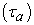peak is not produced and settling time with value as 1.385. The settling time as 1.404, 1.519, 1.557, and peak value as 0.9839, 1.016, 1.023, respectively from -15% to +20% for amplifier time constants. The settling time for the -20% of exciter time constants is 1.385 with no peak amplitude. For the time constant of exciterevaluates the settling time and peak values are 1.461, 1.653, 1.710 and 0.9687, 1.023, 1.031, respectively ranging from -15% to +20%.

For the generator time constantpresents the settling time with values 1.385, 1.404, 1.576, 1.633 and peak with values 0.9686, 0.9768, 1.014, 1.019, respectively between the range of -20% to +20%. The time constants of sensor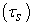obtain the settling time as 1.519, 1.519, 1.500, 1.538 and peak value as 0.9959, 0.9963, 0.9991, 0.9996, respectively in the range of -20% to +20% as mentioned in Table 6.

• Figure 15. Robustness analysis of AVR system by changing time constant ranging from-20% to +20% for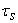In terms of performance indices, evaluates the error functions such as ISE, IAE and ITAE to check the robustness of the AVR system optimized with FPA-PID controller as presented in Table 6. The varying parameterfrom -20% to +20% obtained the ISE values as 19.5244, 19.5270, 19.5396, 19.5418, IAE values as 19.6362, 19.6362, 19.6362, 19.6362 and ITAE values as 199.8600, 199.8618, 199.8726, 199.8744, respectively. The exciter time constantsrepresents the ISE with values 19.5167, 19.5209, 19.5453, 19.5492, IAE with values 19.6363, 19.6363, 19.6362, 19.6362 and ITAE with values 199.8384, 199.8456, 199.8888, 199.8960, respectively.

The time constantobtained the ISE values as 19.5132, 19.5183, 19.5475, 19.5521, IAE values as 19.6364, 19.6363, 19.6361, 19.6361 and ITAE values as 199.7958, 199.8136, 199.9208, 199.9387, respectively between the range -20% to +20% as shown in Table 6. The time constantproduced the ISE with values 19.5301, 19.5309, 19.5356, 19.5364, IAE with values 19.6342, 19.6347, 19.6377, 19.6382 and ITAE with values 199.8657, 199.8661, 199.8683, 199.8687, respectively ranging from -20% to +20 % as enlisted in Table 6.

According to above analysis and simulation results, it is show that the FPA-PID controller is robust in nature.

5. Conclusion

In this work, the FPA optimization technique is considered to effective design of PID controller for AVR system. The optimally tuned FPA-PID controller is take into account using integral absolute error. The performance of proposed algorithm is measured for 100 number of iterations. The AVR system response with proposed FPA-PID controller is found to be better and compared that of with controllers in literature (42 in number). The performance is compared in terms of peak value, settling time and performance indices like ISE, IAE and ITAE. The robustness analysis of the proposed FPA based PID controller is considered for 156 plant conditions and varying time constants parameters as ±20% system conditions. The system stability with proposed FPA optimized PID controller also analyzed in the form of eigenvalues, damping factor and frequency of oscillations.

References

  Memon, A.P., Memon, A.S., Akhund, A.A., and Memon, R.H., “Multilayer Perceptrons Neural Network Automatic Voltage Regulator With Applicability And Improvement In Power System Transient Stability,” International Journal of Emerging Trends in Electrical and Electronics, 9 (0). 30-38. Nov. 2013 2013. In article View Article  Memon, A.P., Uqaili, M.A., Memon, Z.A., and Tanwani, N.K., “Suitable Feedforward Artificial Neural Network Automatic Voltage Regulator for Excitation Control System,” Universal Journal of Electrical and Electronic Engineering, 2 (2). 45-51. 2014. In article View Article  Mohanty, P.K., Sahu, B.K., and Panda, S., “Tuning and Assessment of Proportional–Integral–Derivative Controller for an Automatic Voltage Regulator System Employing Local Unimodal Sampling Algorithm,” Electric Power Components and Systems, 42 (9). 959-969. 2014/07/04 2014. In article View Article  Rao, P.V.G.K., Subramanyam, M.V., and Satyaprasad, K., “Design of internal model control-proportional integral derivative controller with improved filter for disturbance rejection,” Systems Science & Control Engineering, 2 (1). 583-592. 2014/12/01 2014. In article View Article  Mittal, A. and Rai, P., “Performance analysis of conventional controllers for automatic voltage regulator(avr),” International Journal of Latest Trends in Engineering and Technology, 7 (2). July 2016 2016. In article  Bansal, H.O., Sharma, R., and Sheeraman, P.R., “PID Controller Tuning Techniques: A Review,” Journal of Control Engineering and Technology (JCET), 2 (4). 168-176. 2012 In article View Article  Shayeghi, H., Younesi, A., and Hashemi, Y., “Optimal design of a robust discrete parallel FP + FI + FD controller for the Automatic Voltage Regulator system,” International Journal of Electrical Power & Energy Systems, 67. 66-75. 2015. In article View Article  Wong, C.C., Li, S.A., and Wang, H.Y., “Hybrid evolutionary algorithm for PID controller design of AVR system,” Journal of the Chinese Institute of Engineers, 32 (2). 251-264. 2009/03/01 2009. In article View Article  Sambariya, D.K. and Paliwal, D., “Optimal design of PIDA controller using harmony search algorithm for AVR power system,” in 2016 IEEE 6th International Conference on Power Systems (ICPS), 1-6, 2016. In article View Article  Gupta, T. and Sambariya, D.K., “Optimal design of fuzzy logic controller for automatic voltage regulator,” in 2017 International Conference on Information, Communication, Instrumentation and Control (ICICIC), 1-6, 2017. In article View Article  Sambariya, D.K. and Paliwal, D., “Optimal design of PIDA controller using firefly algorithm for AVR power system,” in 2016 International Conference on Computing, Communication and Automation (ICCCA), 987-992, 2016. In article View Article  Panda, S., Sahu, B.K., and Mohanty, P.K., “Design and performance analysis of PID controller for an automatic voltage regulator system using simplified particle swarm optimization,” Journal of the Franklin Institute, 349 (8). 2609-2625. 2012. In article View Article  Sambariya, D.K. and Gupta, T., “Optimal design of PID controller for an AVR system using monarch butterfly optimization,” in 2017 International Conference on Information, Communication, Instrumentation and Control (ICICIC), 1-6, 2017. In article View Article  dos Santos Coelho, L., “Tuning of PID controller for an automatic regulator voltage system using chaotic optimization approach,” Chaos, Solitons & Fractals, 39 (4). 1504-1514. 2009. In article View Article  Yang, X.-S., “Flower pollination algorithm for global optimization,” in UCNC, 240-249, 2012. In article View Article  Chiroma, H., Shuib, N.L.M., Muaz, S.A., Abubakar, A.I., Ila, L.B., and Maitama, J.Z., “A review of the applications of bio-inspired flower pollination algorithm,” Procedia Computer Science, 62. 435-441. 2015. In article View Article  Yang, X.-S., Karamanoglu, M., and He, X., “Flower pollination algorithm: a novel approach for multiobjective optimization,” Engineering Optimization, 46 (9). 1222-1237. 2014. In article View Article  Yang, X.-S., Karamanoglu, M., and He, X., “Multi-objective flower algorithm for optimization,” Procedia Computer Science, 18. 861-868. 2013. In article View Article  Wang, R. and Zhou, Y., “Flower pollination algorithm with dimension by dimension improvement,” Mathematical Problems in Engineering, 2014. 2014. In article View Article  Abdel-Raouf, O. and Abdel-Baset, M., “A new hybrid flower pollination algorithm for solving constrained global optimization problems,” International Journal of Applied Operational Research-An Open Access Journal, 4 (2). 1-13. 2014. In article View Article  Sambariya, D.K. and Paliwal, D., “Optimal design of PIDA controller using Firefly algorithm for AVR power system,” in International Conference on Computing, Communication and Automation (ICCCA-2016), School of Computing Science and Engineering Galgotias University, Greater Noida, UP (INDIA) PIN-201308 1-6, 2016. In article View Article  Afroomand, A., Tavakoli, S., and Tavakoli, M., “An efficient metaheuristic optimization approach to the problem of PID tuning for automatic voltage regulator systems,” in 2016 IEEE International Conference on Advanced Intelligent Mechatronics (AIM), 1682-1687, 2016. In article View Article  Chatterjee, S. and Mukherjee, V., “PID controller for automatic voltage regulator using teaching–learning based optimization technique,” International Journal of Electrical Power & Energy Systems, 77. 418-429. 2016. In article View Article  Verma, S.K., Yadav, S., and Nagar, S.K., “Controlling of an automatic voltage regulator using optimum integer and fractional order PID controller,” in 2015 IEEE Workshop on Computational Intelligence: Theories, Applications and Future Directions (WCI), 1-5, 2015. In article View Article  Narendra Kumar, Y., Panda, S., Tentu, P., and Durgamalleswarao, K., “Comparative analysis of PID controller for an automatic voltage regulator system,” in 2015 International Conference on Electrical, Electronics, Signals, Communication and Optimization (EESCO), 1-6, 2015. In article View Article  Aberbour, J., Graba, M., and Kheldoun, A., “Effect of cost function and PSO topology selection on the optimum design of PID parameters for the AVR System,” in 2015 4th International Conference on Electrical Engineering (ICEE), 1-5, 2015. In article View Article  Gozde, H., Taplamacioğlu, M.C., and Ari, M., “Automatic Voltage Regulator (AVR) design with Chaotic Particle Swarm Optimization,” in Proceedings of the 2014 6th International Conference on Electronics, Computers and Artificial Intelligence (ECAI), 23-26, 2014. In article View Article  Priyambada, S., Mohanty, P.K., and Sahu, B.K., “Automatic voltage regulator using TLBO algorithm optimized PID controller,” in 2014 9th International Conference on Industrial and Information Systems (ICIIS), 1-6, 2014. In article View Article  Hasanien, H.M., “Design Optimization of PID Controller in Automatic Voltage Regulator System Using Taguchi Combined Genetic Algorithm Method,” IEEE Systems Journal, 7 (4). 825-831. 2013. In article View Article  Nirmal, J.F. and Auxillia, D.J., “Adaptive PSO based tuning of PID controller for an Automatic Voltage Regulator system,” in 2013 International Conference on Circuits, Power and Computing Technologies (ICCPCT), 661-666, 2013. In article View Article  Kumar, A. and Gupta, D.R., “Compare the results of Tuning of PID controller by using PSO and GA Technique for AVR system,” International Journal of Advanced Research in Computer Engineering & Technology (IJARCET), 2 (6). 2130-2138. 2013. In article View Article  Bayram, M.B., Bülbül, H.İ., Can, C., and Bayindir, R., “Matlab/GUI based basic design principles of PID controller in AVR,” in 4th International Conference on Power Engineering, Energy and Electrical Drives, 1017-1022, 2013. In article View Article  Shayeghi, H. and Dadashpour, J., “Anarchic Society Optimization Based PID Control of an Automatic Voltage Regulator (AVR) System,” Electrical and Electronic Engineering, 2 (4). 199-207. 2012. In article View Article  Sahu, B.K., Mohanty, P.K., Panda, S., Kar, S.K., and Mishra, N., “Design and comparative performance analysis of PID controlled automatic voltage regulator tuned by many optimizing liaisons,” in 2012 International Conference on Advances in Power Conversion and Energy Technologies (APCET), 1-6, 2012. In article View Article  PubMed  Sahu, B.K., Panda, S., Mohanty, P.K., and Mishra, N., “Robust analysis and design of PID controlled AVR system using Pattern Search algorithm,” in 2012 IEEE International Conference on Power Electronics, Drives and Energy Systems (PEDES), 1-6, 2012. In article View Article  PubMed  Mandal, A., Zafar, H., Ghosh, P., Das, S., and Abraham, A., “An efficient memetic algorithm for parameter tuning of PID controller in AVR system,” in 2011 11th International Conference on Hybrid Intelligent Systems (HIS), 265-270, 2011. In article View Article  Rahimian, M. and Raahemifar, K., “Optimal PID controller design for AVR system using particle swarm optimization algorithm,” in 2011 24th Canadian Conference on Electrical and Computer Engineering(CCECE), 000337-000340, 2011. In article View Article  Madinehi, N., Shaloudegi, K., Abedi, M., and Abyaneh, H.A., “Optimum design of PID controller in AVR system using intelligent methods,” in 2011 IEEE Trondheim PowerTech, 1-6, 2011. In article View Article  Zhu, H., Li, L., Zhao, Y., Guo, Y., and Yang, Y., “CAS algorithm-based optimum design of PID controller in AVR system,” Chaos, Solitons & Fractals, 42 (2). 792-800. 2009. In article View Article  Zwe-Lee, G., “A particle swarm optimization approach for optimum design of PID controller in AVR system,” IEEE Transactions on Energy Conversion, 19 (2). 384-391. 2004. In article View Article  Kumar, A. and Shankar, G., “Priority based optimization of PID controller for automatic voltage regulator system using gravitational search algorithm,” in 2015 International Conference on Recent Developments in Control, Automation and Power Engineering (RDCAPE), 292-297, 2015. In article View Article

Published with license by Science and Education Publishing, Copyright © 2018 D. K. Sambariya and Tripti GuptaThis work is licensed under a Creative Commons Attribution 4.0 International License. To view a copy of this license, visit http://creativecommons.org/licenses/by/4.0/